### Math History

"Math" is an enormous and almost entirely unknown wilderness of patterns, ideas, and truths. If anyone has led you to believe otherwise, they lied.If textbook authors were responsible cartographers, there would be notes on almost every page reminding people that no one has ever traveled to at least $99\%$ of this planet's surface.

# Mathematical Stories

So, you don't have to travel $1000$ miles studying mathematics in school to get into uncharted territory. In fact, in each of the histories in this course, the questions that the mathematician researched bridge from ancient tools to modern, unanswered research topics.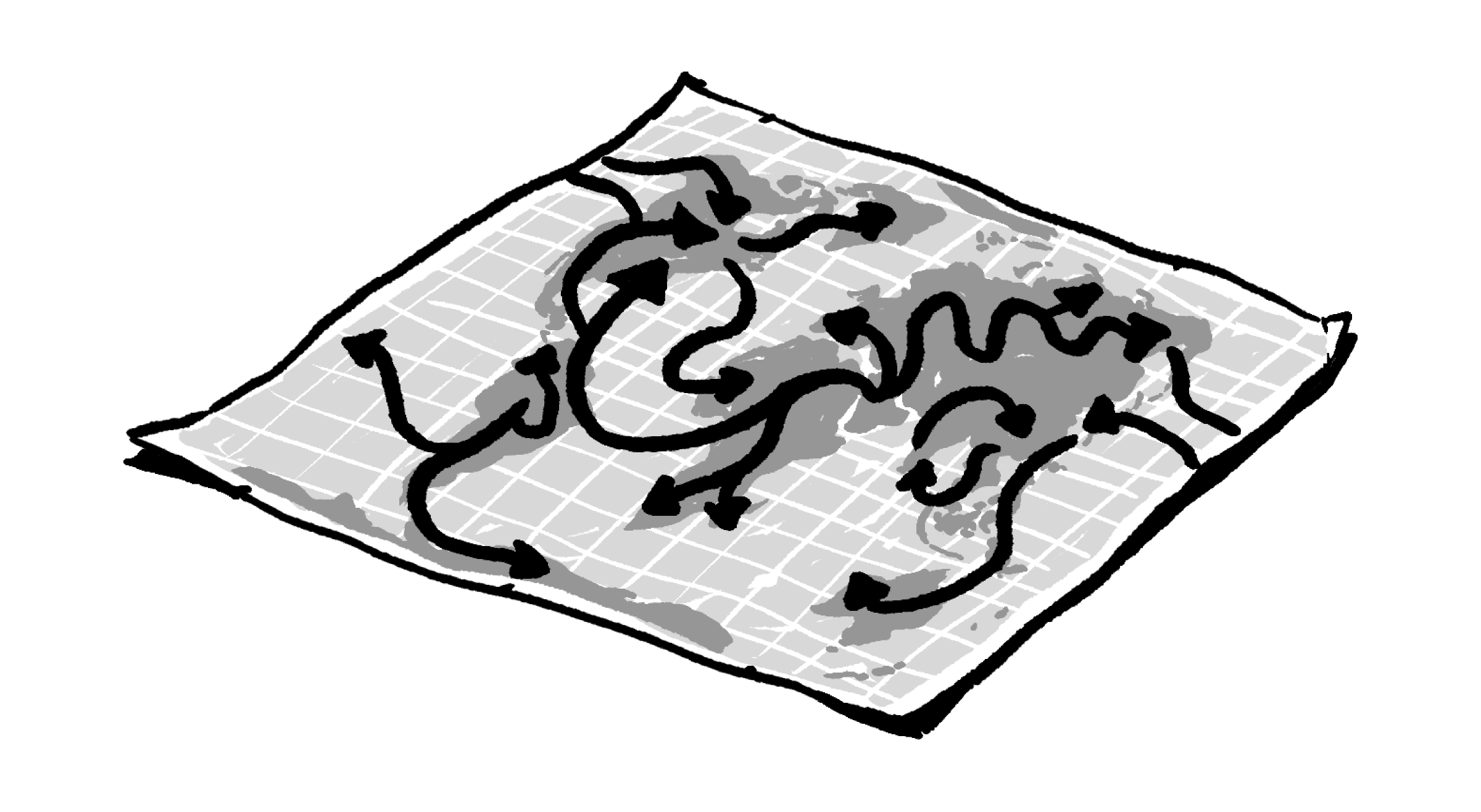# Mathematical Stories

And you're not going to just sit back and watch. You might be able to enjoy the story alone that way, but mathematics is best understood and appreciated when you actually dig in and solve problems.

For example, here is a pattern of numbers with a rule that determines its values: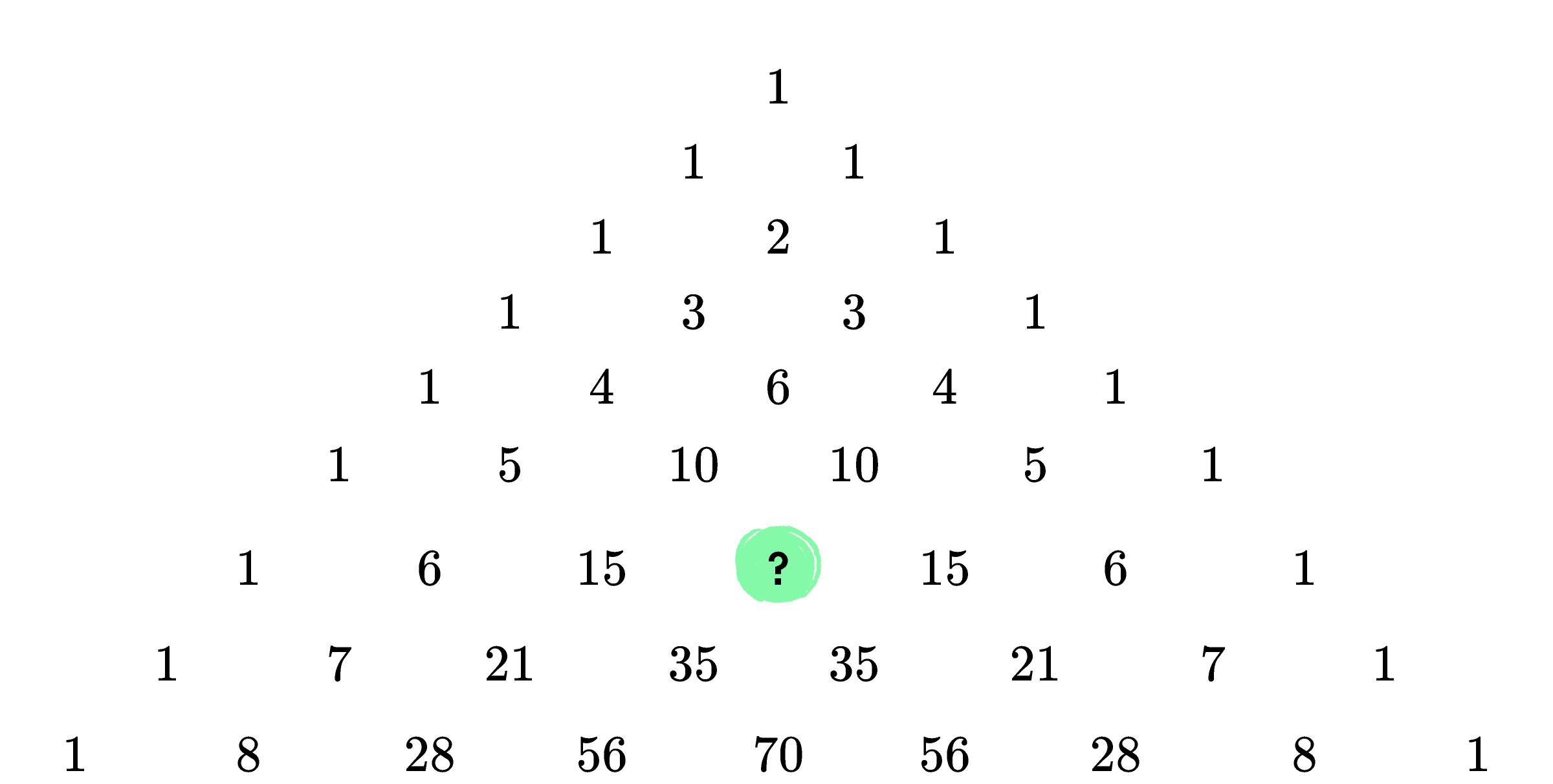What is the missing number?

# Mathematical Stories

This mathematical object can be constructed from a simple rule (every number in the triangle is the sum of the two numbers above it), but it contains a remarkable number of different patterns.

Let’s look at one of the patterns. When we look at sums of the horizontal rows, we can see that each row's sum is larger than the one above it, and they grow in a predictable way: $1, 2, 4, 8, 16, \dots.$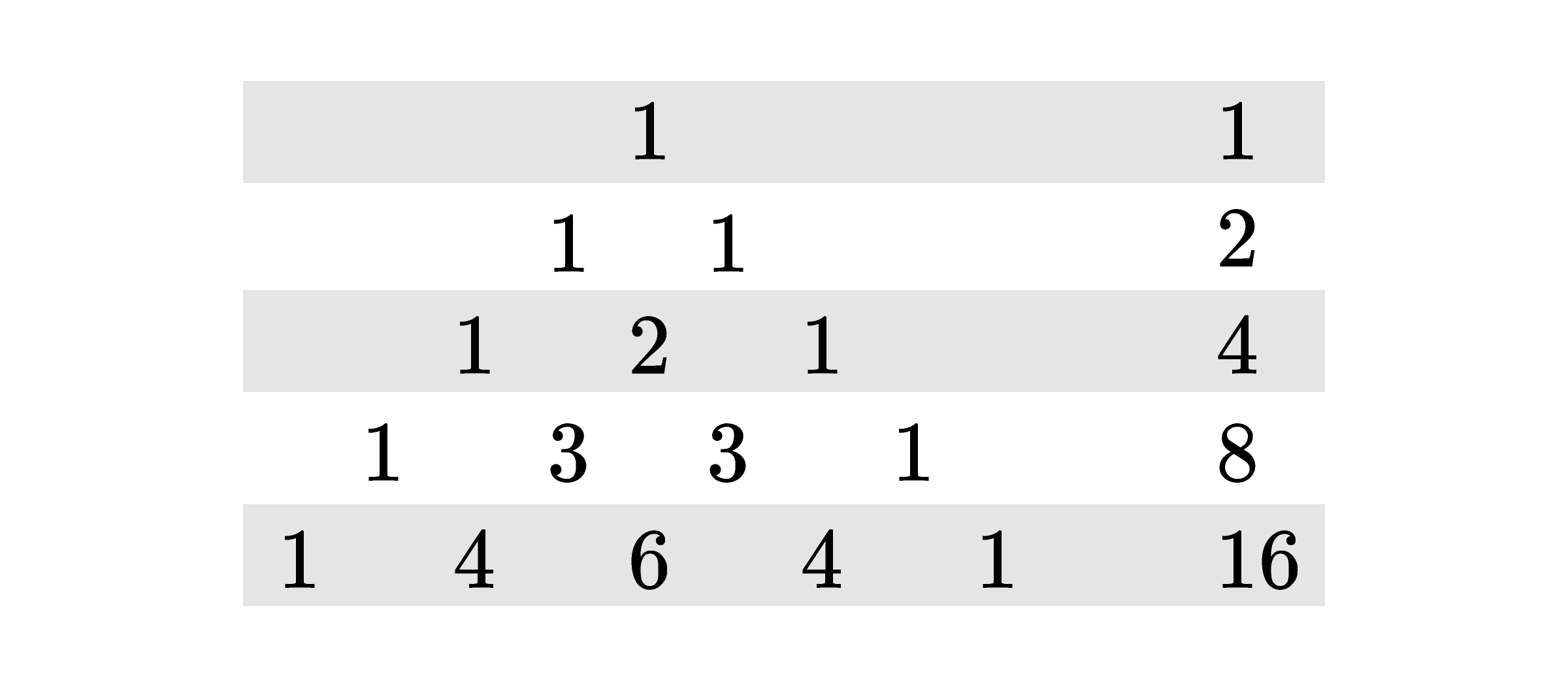As we can see above, the row that begins $1, 4, 6, \dots$ has a sum of $16.$ What is the sum of the row that begins $1, 9, 36, \dots ?$

# Mathematical Stories

We might consider adding up the numbers in a slightly different way. By shifting the triangle and slanting the rows a bit, we can find a different set of sums: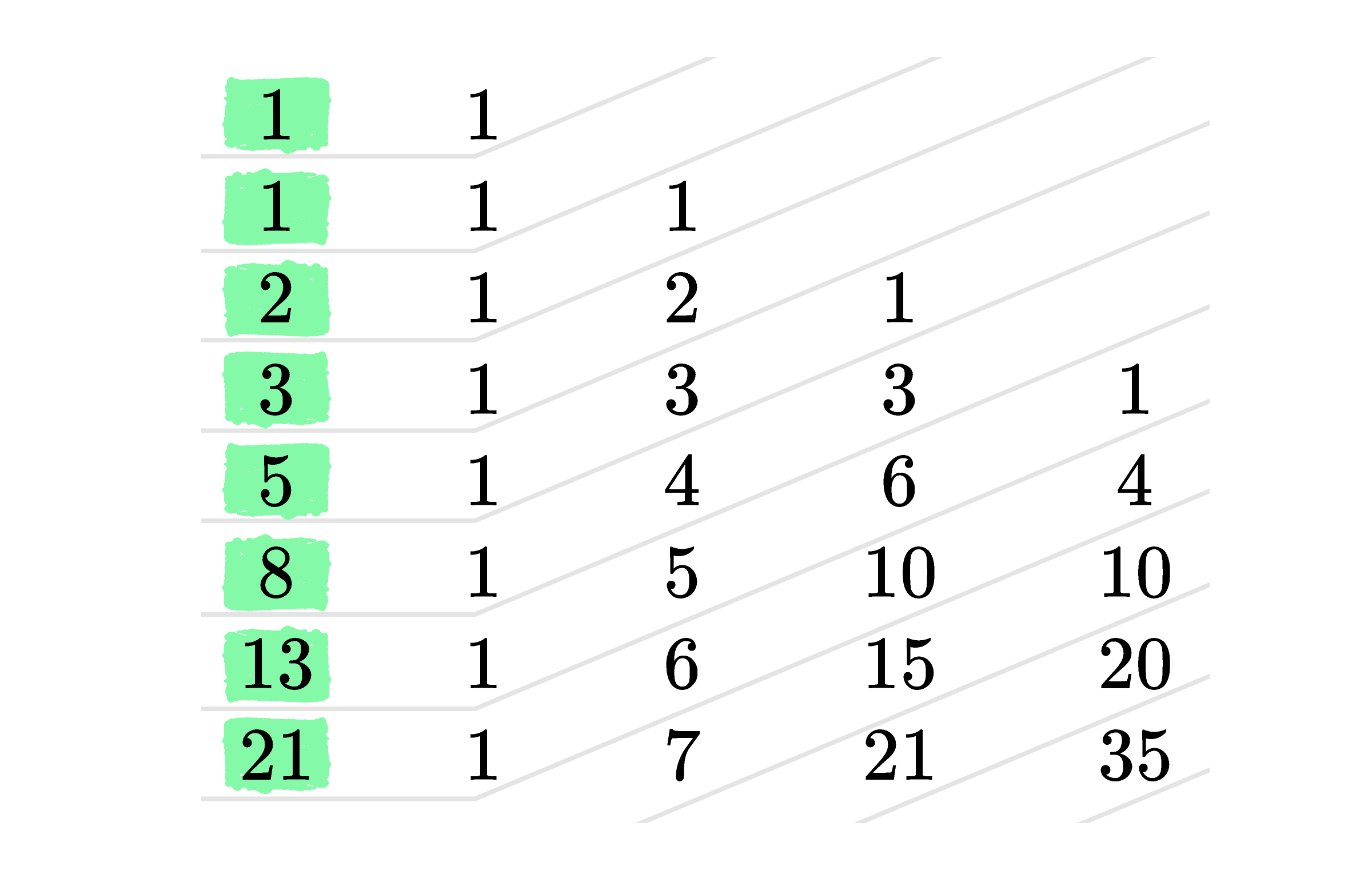The slanted row sums are $1, 1, 2, 3, 5, 8, 13, 21, \ldots.$ What's the next slanted row sum after $21?$

# Mathematical Stories

Not only are there interesting sequences hidden in the triangle, but also there is a bit of surprising algebra. Consider the binomial $(a+b)^n$ for different powers of $n.$

If you look closely at the coefficients of the binomial’s expansion, you can see a relationship to the numbers in the triangle.

What is the coefficient of the $a^3b^3$ term in the expansion of $(a+b)^6?$# Mathematical Stories

To wrap up our investigation of this object (for now), let’s look at what happens when we shade all the odd numbers in the triangle.

# Mathematical Stories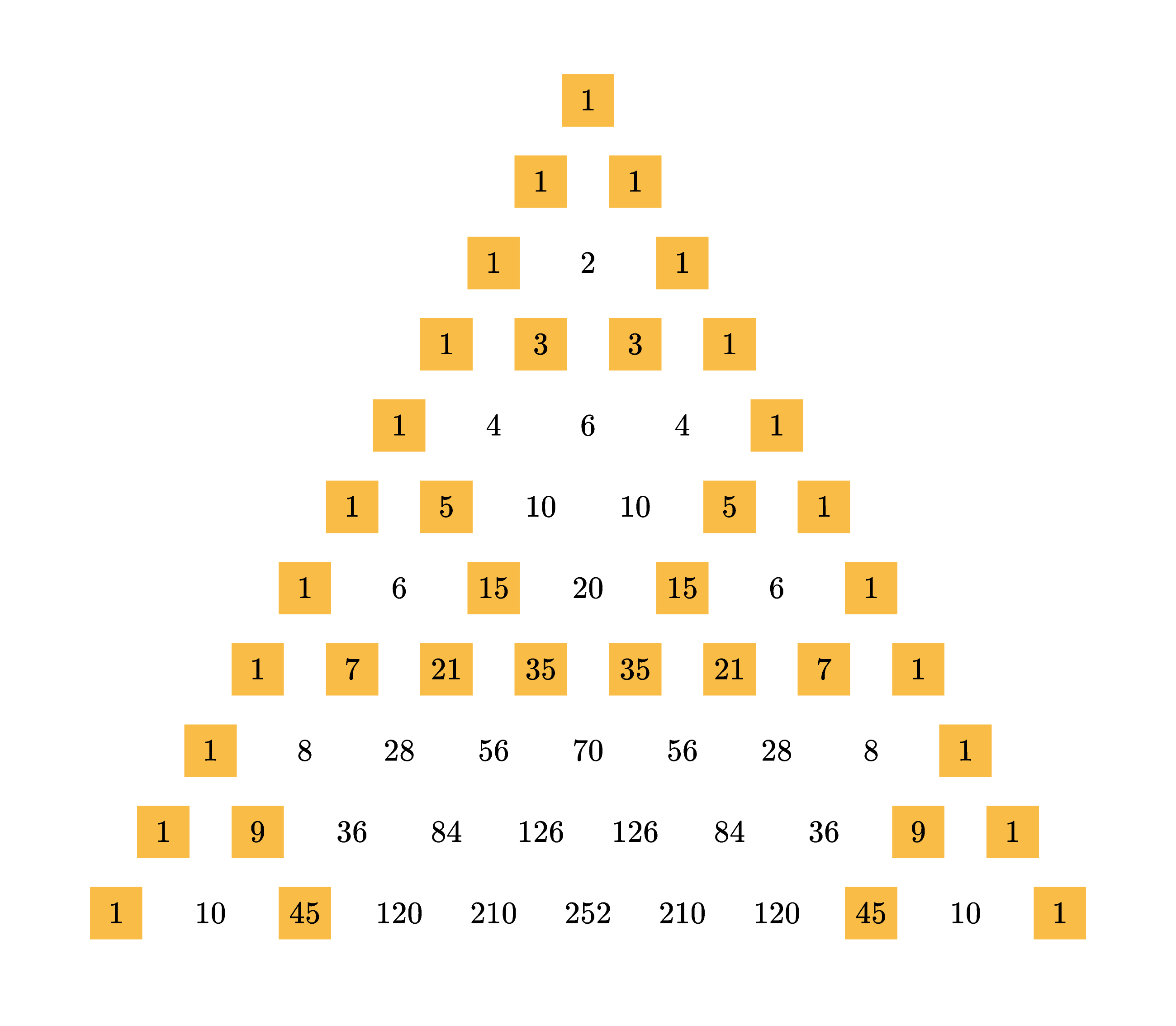The image above shows what happens when the odd values of the triangle are shaded in. How many odd numbers are there in the row that begins $1, 15, \dots ?$

# Mathematical Stories

If you were surprised to find an infinitely complex fractal pattern hiding in a triangle formed by a simple adding rule, you are not alone.

This shape has been a source of fascination for thousands of mathematicians across many cultures and centuries. Although much has been learned about it, there are still far more questions than answers about this triangle. For example, we still don't have any good idea about how to classify how often most numbers appear in the triangle.

The current record holder is $3003,$ which appears eight times in the triangle. Other than $1$ appearing infinitely many times, no other number has been found in the triangle more than six times. No one knows if $3003$ is special or if there are millions of other numbers that appear that often (or more).

And there are thousands of questions like that and infinitely more that no one has ever even asked.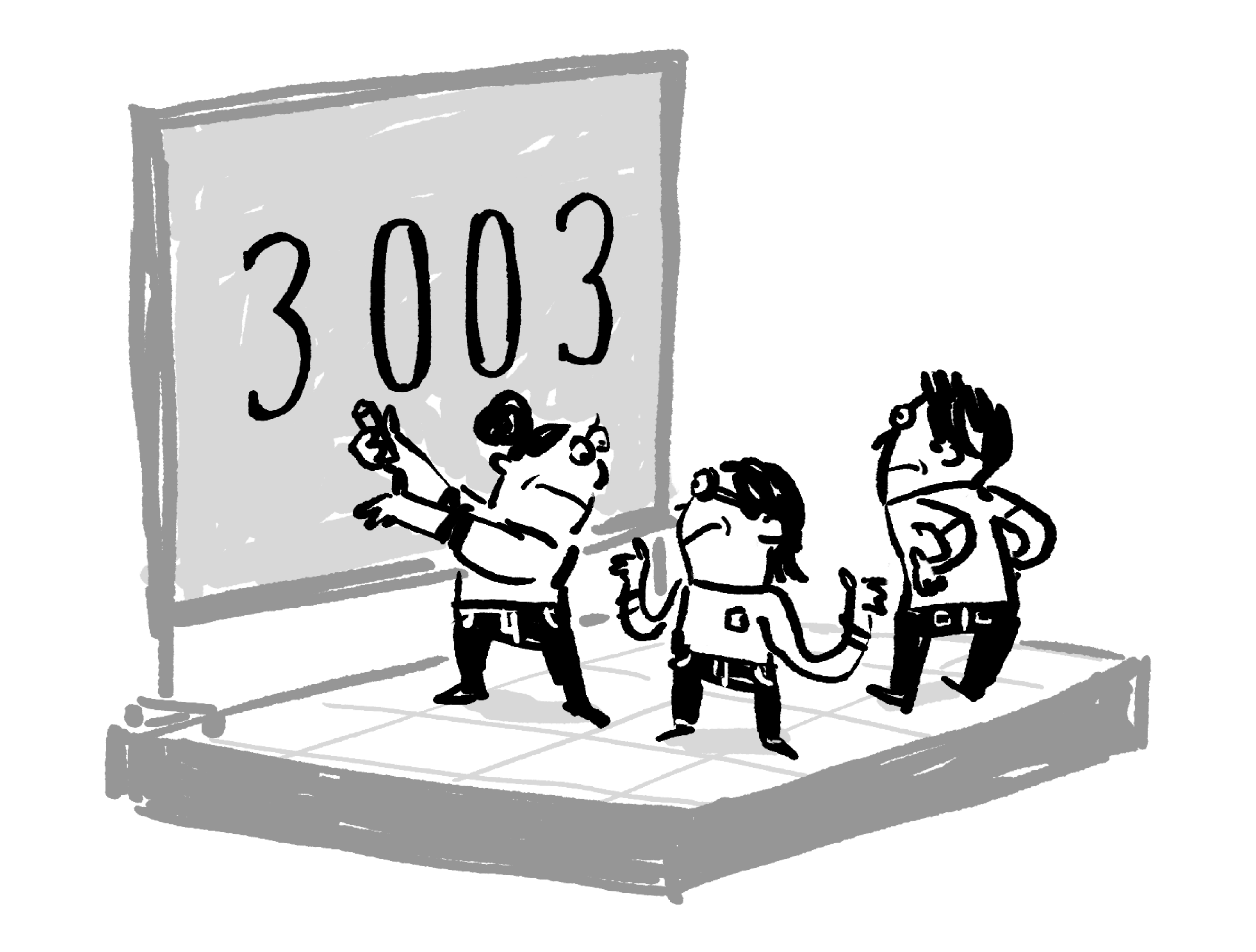# Mathematical Stories

Hopefully, seeing a tiny sliver of the complexity in this one simple pattern helps you see how much room there is in mathematics and why only a tiny bit of that room is well mapped out.

Now that you're a little familiar with this pattern, let's talk about its history. It's not a straightforward story.

There are mentions of some of the properties of this triangle dating back to at least the second century B.C., but all that survives about the triangle from those days are fragments. Our first solid record of this figure is from India, dating from around $975$ A.D.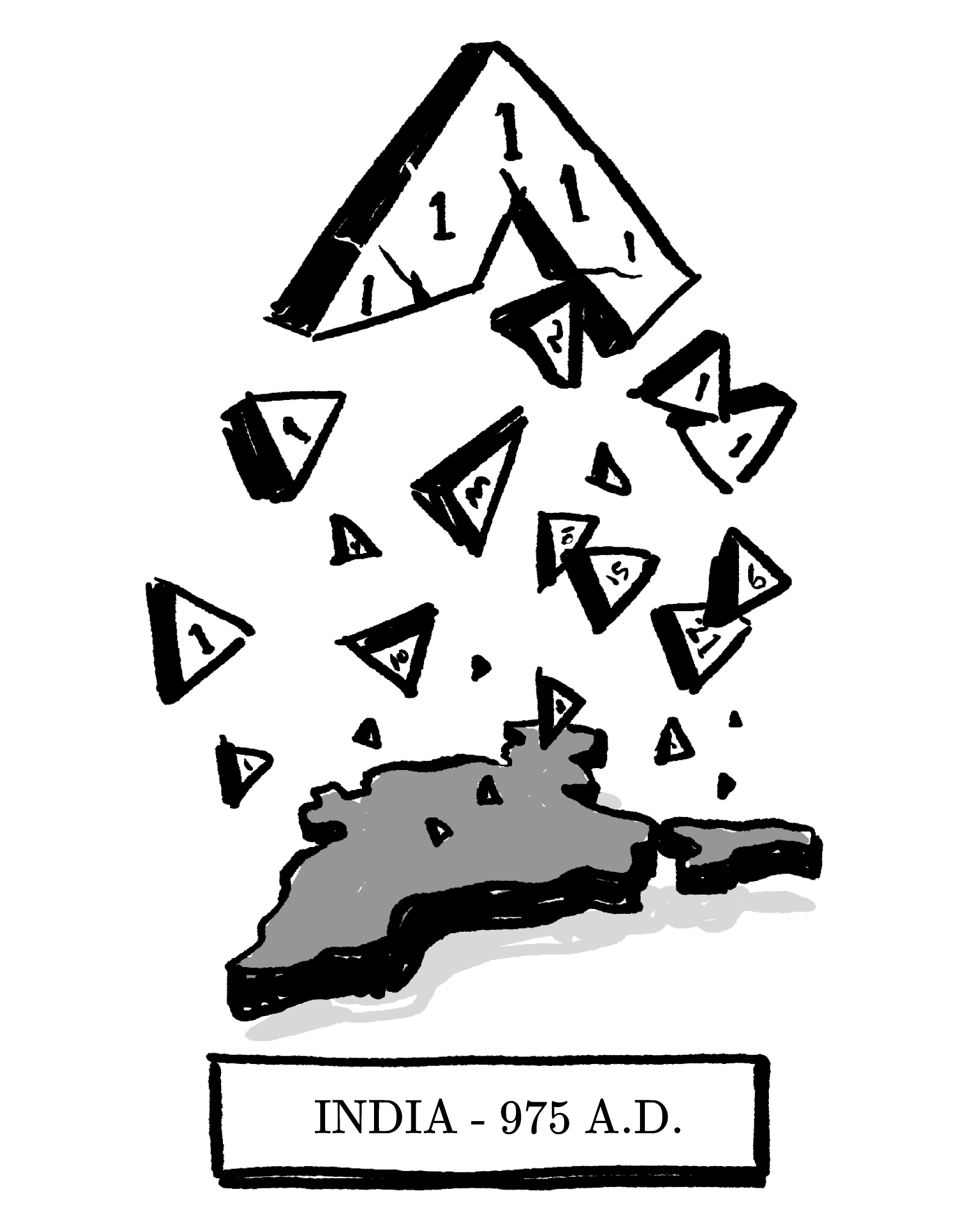# Mathematical Stories

While the triangle is well-known and has been studied for centuries, it isn't known by the same name everywhere, not even today.

In the oldest known surviving record of this triangle $($from India circa $975$ AD$),$ it’s called Meru-prastaara, the Staircase of Mount Meru.

• In Iran, it’s referred to as Khayyam’s Triangle.
• In Italy, it's called Tartaglia’s Triangle.
• In most of the English-speaking Western world, its name is Pascal’s Triangle.
• And it's Yang Hui's Triangle in China.

These are the names of mathematicians, and they all contributed to what we now know about this triangle — but not one of them discovered it.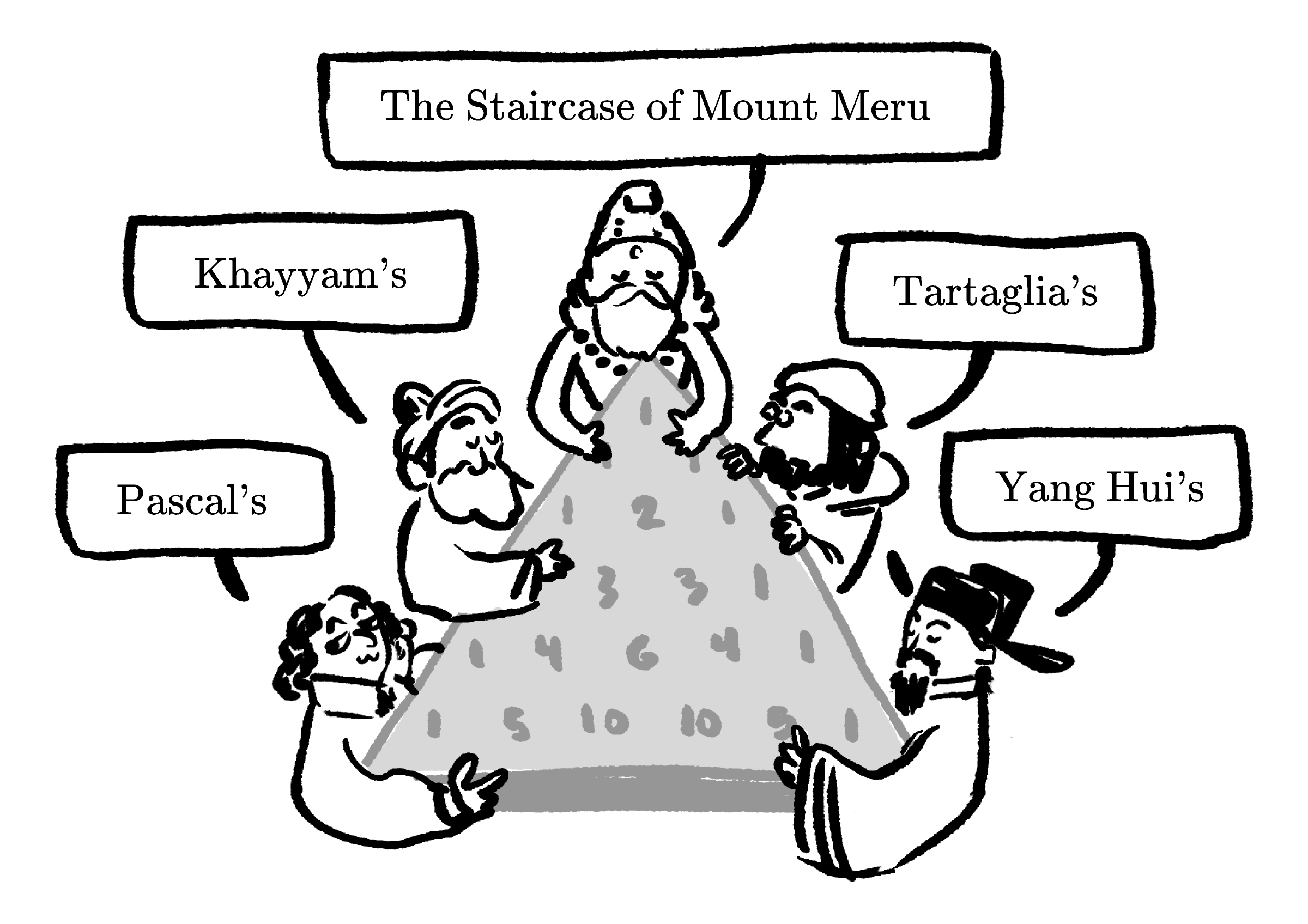# Mathematical Stories

In truth, the question of who discovered this pattern doesn't have one single answer. Many people have worked on it independently and, over thousands of years, the accumulated knowledge we have about it can't be attributed to any one person or culture.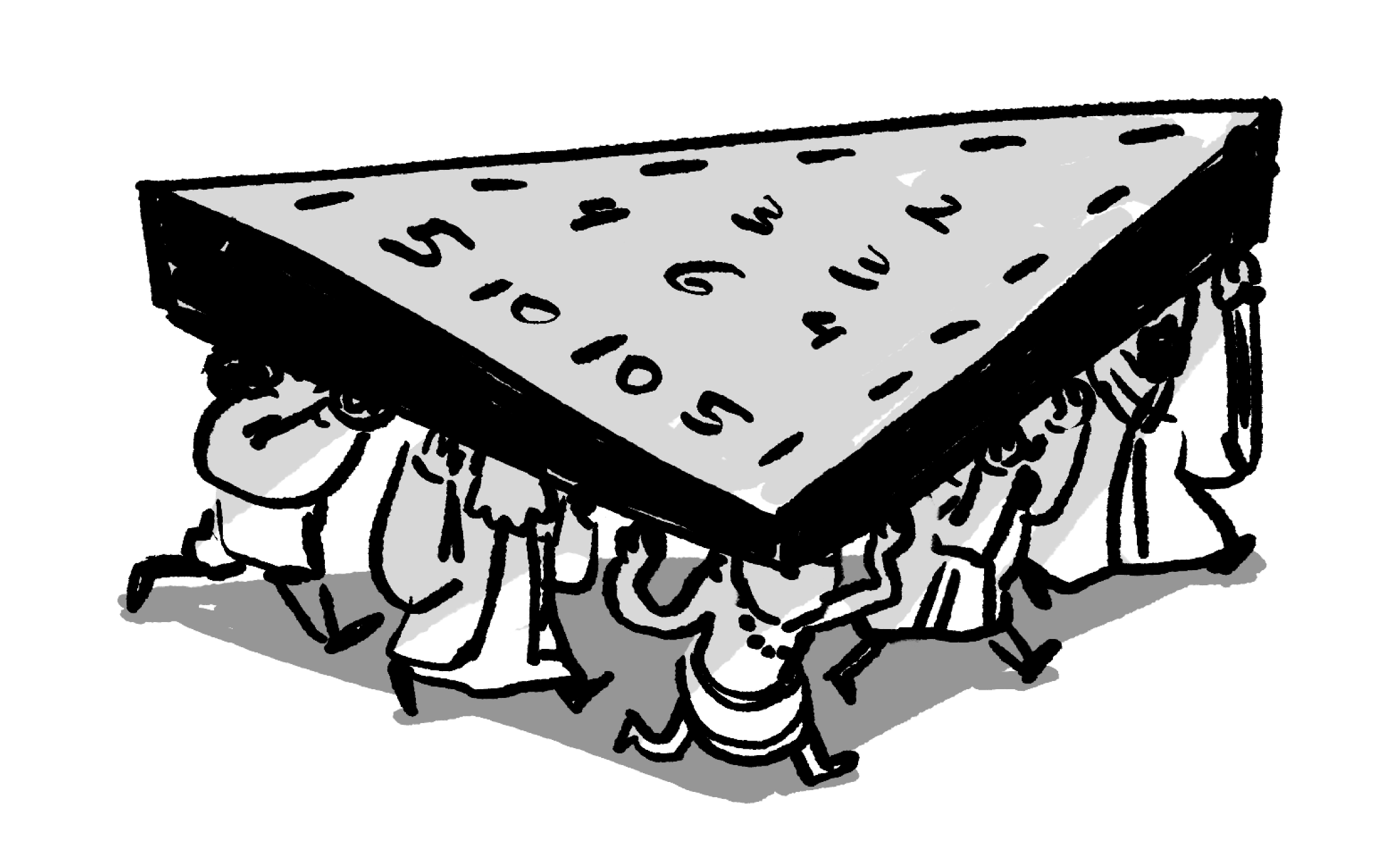# Mathematical Stories

The point is this: there are thousands of people who have been a part of building and mapping mathematics, and we can't tell all their stories. In many cases, no one even knows where an idea truly came from.

And sometimes the person who now gets credit for a piece of mathematics actually had little — or nothing — to do with its creation.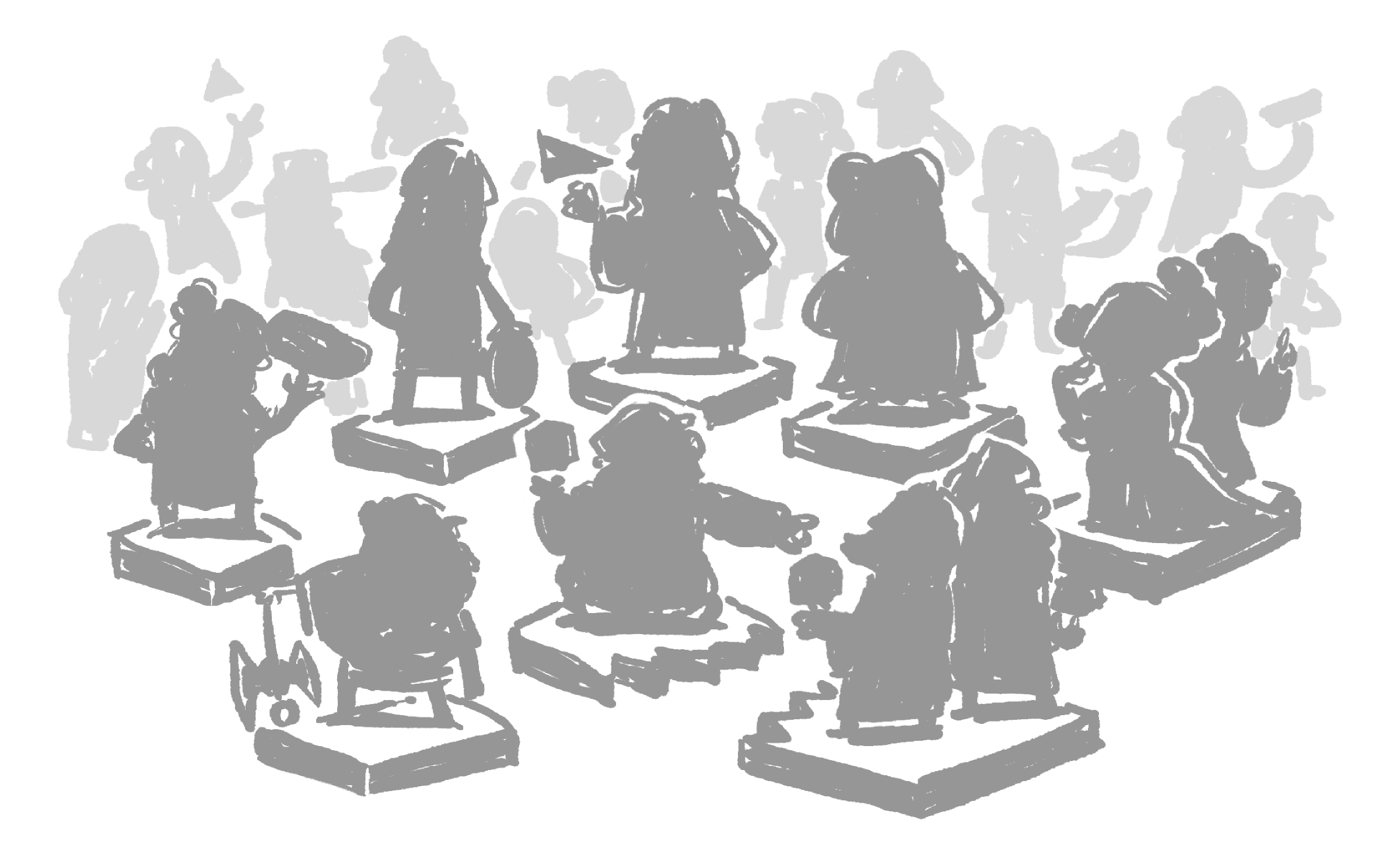# Mathematical Stories

In this course, we’re only able to focus our attention on just a small handful of mathematicians from among the many, many people involved in the long history of mapping the world of math.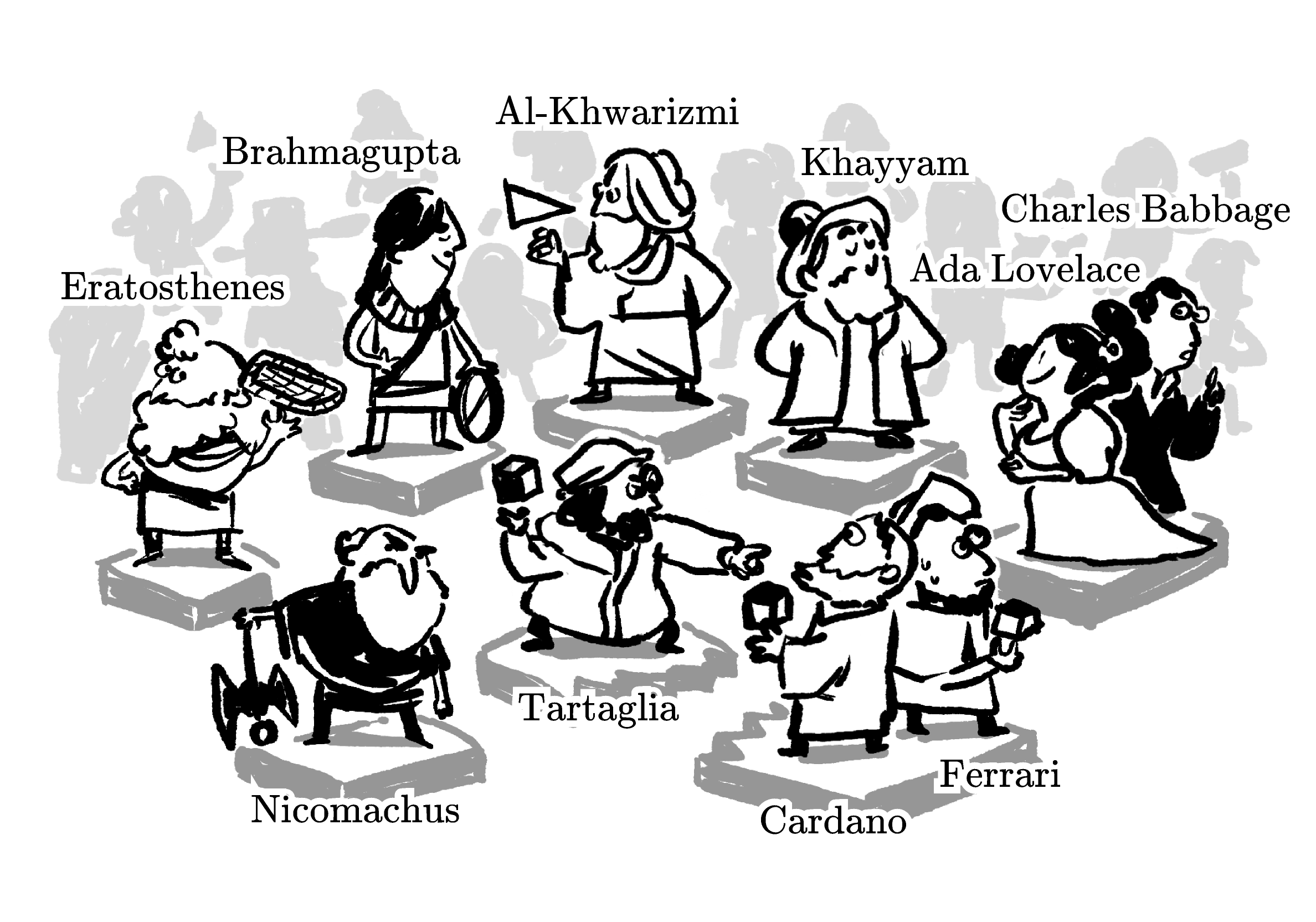It’s not possible for us to see things exactly how these people did since we have the advantage of mathematical tools that they didn’t have.

To make it easier to tell and appreciate these stories, at times we will use some modern conventions — for example, algebraic notation — that weren’t around in those times.

# Mathematical Stories

We're telling stories about complicated, real people and the beautiful and complicated mathematics they studied. Each story is a path through known and unknown territory.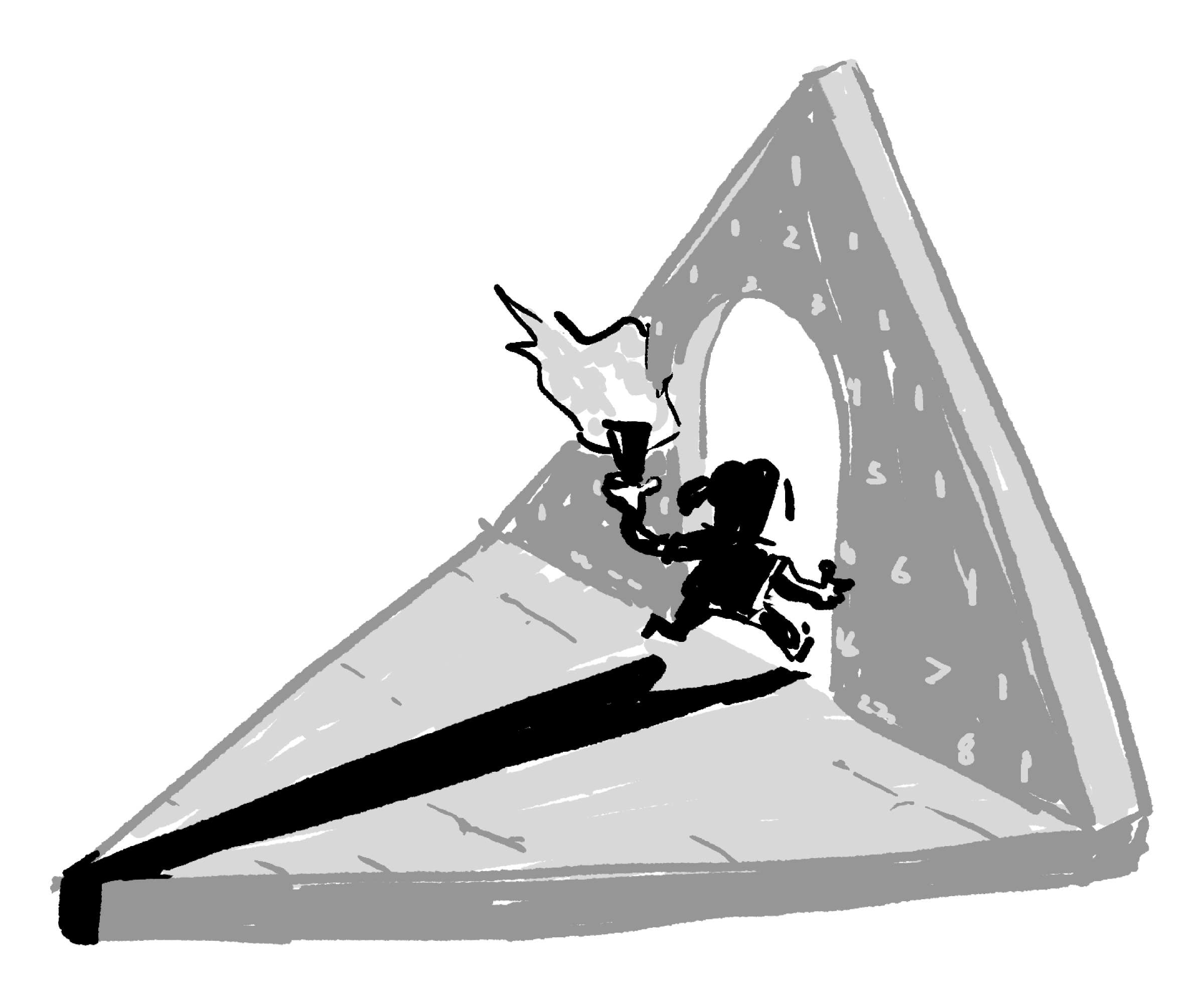What you'll see in the shapes of these paths and stories is that, like us, the people in these stories were fascinated by numbers, by calculation, and by the surprising patterns that can be found in mathematics.

# Mathematical Stories

×